Worksheet: Addition And Subtraction Up To 99

# Worksheet: Addition And Subtraction Up To 99 - Notes | Study Mathematics for Class 1 - Class 1

 1 Crore+ students have signed up on EduRev. Have you?

Question 1:

(a)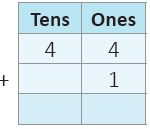(b)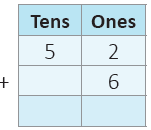(c)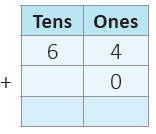Question 2:

(a)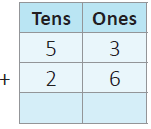(b)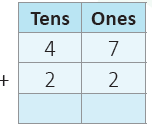(c)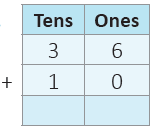Subtract the following.

Question 3:
(a)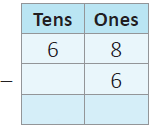(b)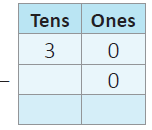(c)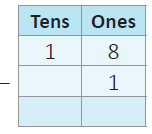Question 4:
(a)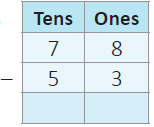(b)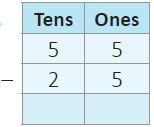(c)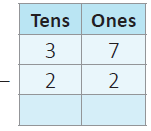Question 5: Subtract by splitting numbers in your notebook.

(a) 24 from 78
(b) 57 from 98
(c) 21 from 69

(d) 46 from 58

(a) 80 and 11

(b) 40 and 50

(c) 46 and 22

(d) 66 and 33

Question 7: Represent the following addition or subtraction sums using expanded form. One is done for you.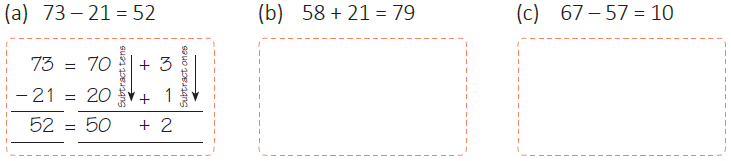Question 8: Addition and Subtraction Stories in Real Life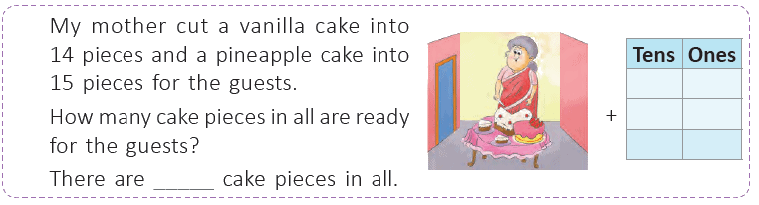The document Worksheet: Addition And Subtraction Up To 99 - Notes | Study Mathematics for Class 1 - Class 1 is a part of the Class 1 Course Mathematics for Class 1.
All you need of Class 1 at this link: Class 1

## Mathematics for Class 1

10 videos|36 docs|23 tests
 Use Code STAYHOME200 and get INR 200 additional OFF

## Mathematics for Class 1

10 videos|36 docs|23 tests

Track your progress, build streaks, highlight & save important lessons and more!

,

,

,

,

,

,

,

,

,

,

,

,

,

,

,

,

,

,

,

,

,

;